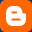## Sunday, January 8, 2012

### Python Tutorial 2: Uderstanding Variables, Operators etc...

This is Python tutorial no. 2
One thing you should note here is '>>>' in the tutorials is the python
prompt which  implies that python interpreter is ready to interpret your

commands and '...' is padded  with multiline statements by python shell,
they are not the part of input or output.So  if you copy any examples from
here don't copy the '>>>' and '...'

The topics covered in this tutorial are :

1.Program input/output(often referred as I/O).

2.Operators

3.Variables

4.Indentation

# 1. Program I/O (if u remember this is a python comment... :)  )
First we should learn about I/O which is basically everywhere
even in the Hello world  program that we created in tutorial 1.

For output we use the print statement like this:

>>> print "Hi this is the output!"

You can use print with string format operator (%), it behaves exactly
like C's printf() function.

>>> print "%s is number %d" % ("Python",1)       # s for string and d                                                                             # for integer

and for input we use the raw_input() .... we use it like this:

>>>x=raw_input("Enter the value of x:")         # here x is a variable.
>>>x                                                            # if u write x and press enter
'2'                                                                 # it shows the value of x.
>>> print  "The value of x is", x                     # you can print variables
#
by separating them with comma.

Note: You can use input() to take input too in Python 2.x but it poses
a security risk and it should not be used.
In Python 3.x raw_input() has been renamed as input() and the
old  input() is no longer there in Python 3.x.

# 2.Operators

Mathematical operators:
+  ----> Addition        Usage :  c=a+b    # a and b should not be mixed
#   types.
#  a=4 and b='string'
#  c=a+b  gives errror!!
-  ----> Subtraction     Usage : c=a-b      # same with subtraction.

*  ----> Multiplication  Usage : c=a*b
/  ----> Division            Usage : c=a/b
%  ----> Modulus/Remainder Usage : c=a%b        # Gives remainder of a/b
# works with integer
#
and floating numbers both.

** ----> Exponentiation  Usage : c=a**b   # gives a  raised to power b.

Comparison operators:
<   ----> Less than  Usage : c=a<b       # Gives True when a is less than b
# otherwise False

<=  ----> Less than or equal to  Usage : c= (a<=b)  # Gives True if a is
# less than or equal to b.

>   ----> Greater than Usage : c=a>b          # Gives True if a is greater than b.
# False otherwise.

>=  ----> Greater than or equal to  Usage: c=(a>=b)        # Gives True
# when a is greater
#  than or equal to
# b. False otherwise.

==  ----> Equal to operator  Usage : c= (a==b)          # Gives True is a is
#
equal to b.
# False otherwise.

=  ----> Assignment  Usage : c=b        # Assigns value of b to c.

!= ----> Not equal to  Usage : c=(a!=b)      # Gives True if a is not equal to b.

Expression conjunction operators:

and ----> and operator Usage: c= a and b         # Gives True if both a and b
# are
both True. False
# otherwise.

or ----> or operator   Usage: c= a or b          # Gives True if both a and b are
# True. False otherwise.

not ----> not operator  Usage: c= not a          # Reverse the values of a.

Python does not have the increment (++n ) or decrement (--n) operator.

# 3.Variables

Rules for naming variables are simple:
1. Characters must be alphanumeric or underscore.
2. First character must not be a digit.

Python is case sensitive.So, var and VAR are two different variables.
Python is dynamically typed. So, you don't have to declare the varibles.

These are some declarations:

>>> x=2
>>> x_9 = 23                       # Varible name with all possible characters.
>>> stri="This is a string "    # a string
>>> 9gag=78                      # First character must not be a digit.
SyntaxError: Invalid syntax

# 4.Indentation

Python uses indentation rather than curly braces. This increases readability
and it clearly identifies a block of code a statement belongs to.

This may seem a bit odd at the beginning but you may like it after some
time.Also, use either Tabs or spaces to indent the code. Mixing both can also
lead to indentation error..

So that's it for this tutorial....Share it with your friends if you liked it...
Next tutorial covers Python Types, Tuple, List, Dictionaries etc...1 CommentsComments
Sergey said...

If you’re looking for a destination to outsource your software development, then one of the Eastern European countries may be a a good match for you. Check out this article to find how to find blockchain developers.